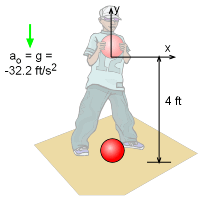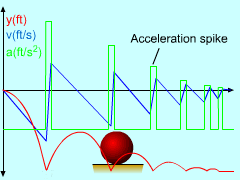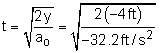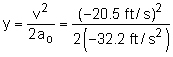Ch 1. Particle General Motion Multimedia Engineering Dynamics Position,Vel & Accel. Accel. varyw/ Time Accel. Constant Rect. Coordinates Norm/Tang. Coordinates Polar Coordinates RelativeMotion
 Chapter - Particle - 1. General Motion 2. Force & Accel. 3. Energy 4. Momentum - Rigid Body - 5. General Motion 6. Force & Accel. 7. Energy 8. Momentum 9. 3-D Motion 10. Vibrations Appendix Basic Math Units Basic Equations Sections Search eBooks Dynamics Fluids Math Mechanics Statics Thermodynamics Author(s): Kurt Gramoll ©Kurt GramollDYNAMICS - CASE STUDY SOLUTION Solution of a)Ball DiagramAcceleration, Velocity and Position Graph Begin by drawing a diagram of the ball at the moment it is released. The origin of the reference axis is fixed at the point where the ball is released, thus      yo = 0     vo = 0     to = 0      y = -4 ft (final position when it hits the ground)      ao = g = -32.2 ft/s2 To determine the velocity when the ball impacts the earth, the time it takes to travel the 4 ft first needs to be calculated.      x(t) = xo + vo (t - to) + 1/2 ao (t - to)2t = 0.498 s The time can now be substituted into the velocity equation,      v = vo + ao (t - to)         = (-32.2 ft/s2) (0.498 s)      v = -16.0 ft/s = -10.9 mi/hr The negative represents the ball traveling downward in the negative y direction. Solution of b) The third equation in the theory section can be used to determined height of the ball so that at impact it is traveling 14 mph. It is assumed that the ball velocity at release is zero.      -14 mi/hr = -20.5 ft/s      v2 = vo2 + 2 ao (y - yo)= -6.55 ft This indicates that when released from rest, the ball will travel 6.55 ft in the negative y direction before its speed is 14 mph. Thus, the ball and the origin of the reference axis must be raised 6.55 ft above the ground.

Practice Homework and Test problems now available in the 'Eng Dynamics' mobile app
Includes over 400 problems with complete detailed solutions.
Available now at the Google Play Store and Apple App Store.